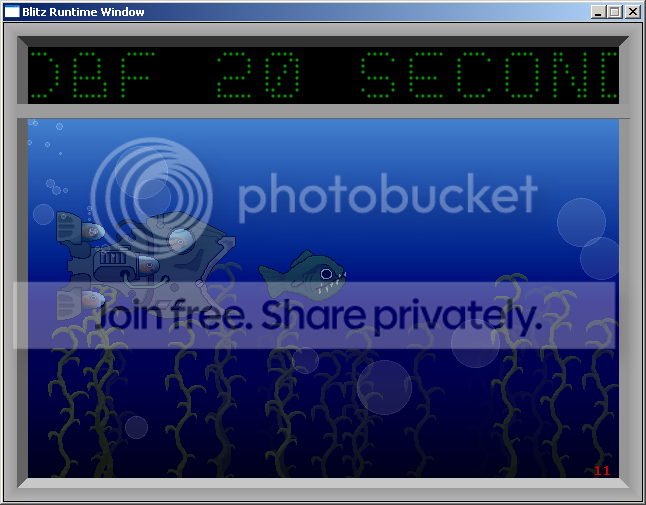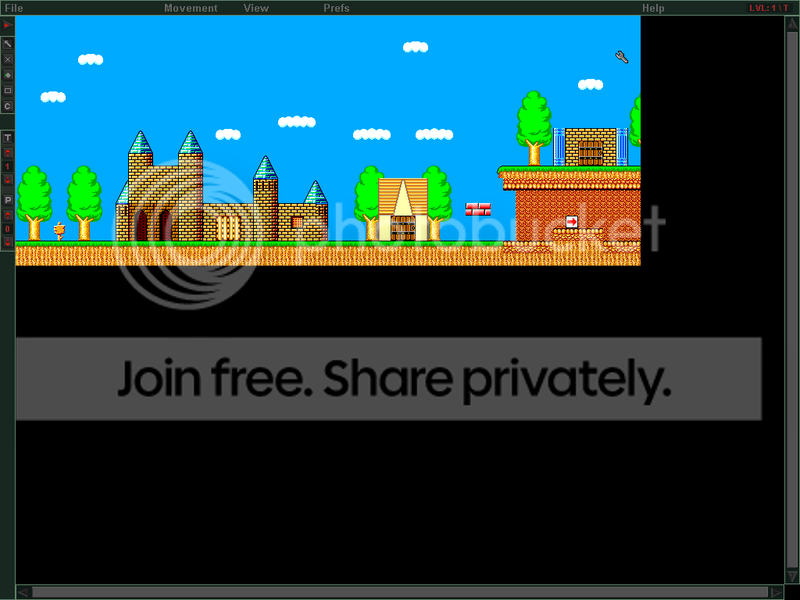-=+=- -=+=- -=+=- -=+=- -=+=- -=+=- -=+=- -=+=- -=+=- -=+=- -=+=- -=+=- -=+=- -=+=- -=+=- -=+=- -=+=- -=+=- -=+=- -=+=- -=+=- -=+=- -=+=- -=+=- -=+=- -=+=- -=+=- -=+=- -=+=- -=+=- (c) WidthPadding Industries 1987 0|710|0 -=+=- -=+=- -=+=- -=+=- -=+=- -=+=- -=+=- -=+=- -=+=- -=+=- -=+=- -=+=- -=+=- -=+=- -=+=- -=+=- -=+=- -=+=- -=+=- -=+=- -=+=- -=+=- -=+=- -=+=- -=+=- -=+=- -=+=- -=+=- -=+=- -=+=-

mike_g# Lavapit Demo

21st August 2007Heres a demo with bouncing fireballs and stuff. It could do with some sound, but other than that its complete.

mike_g# 20 Second Fish Tank Demo

12th March 2007A short old school demo I made. Can anyone let us know if you get a MAV when you run this? It works fine for me but someone else said that they had a problem with it. Cheers :)

mike_g# Space Balls

7th January 2007A Fast Paced 2D Shooter

mike_g# Tile Up

25th September 20062d Tile editor With lots of features

More - Older Posts